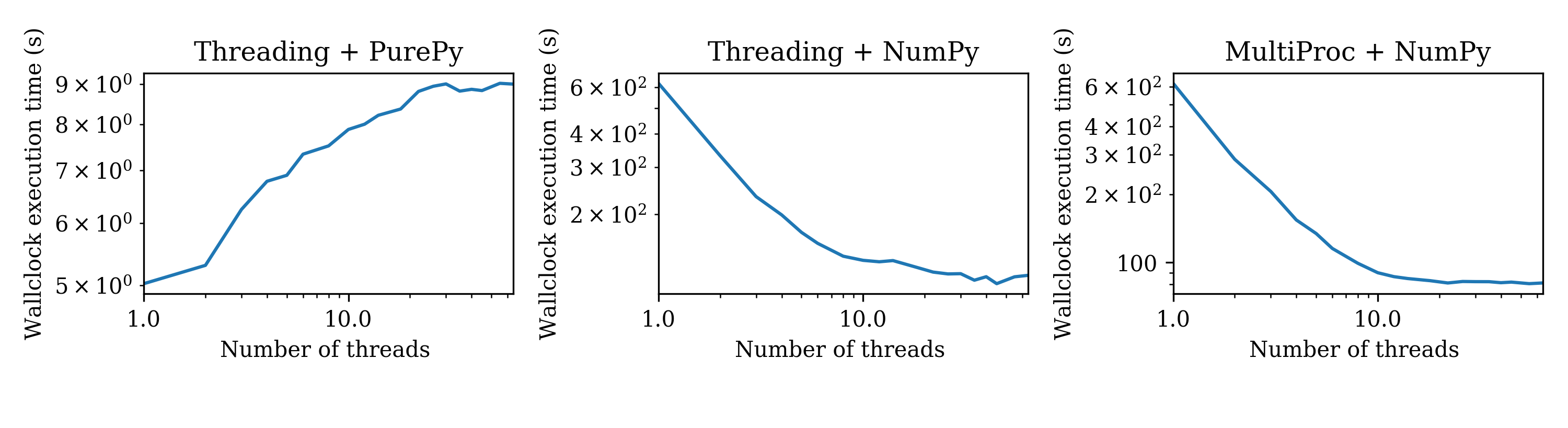# Python Global Interpreter Lock (GIL) + numerical data processing

The global interpreter lock may naively seem to imply that a Python process can only use one core of a processor. That is not at all the case: one (well-known) reason is that Python extension code (such as ubiquitous NumPy/SciPy kernels) can themselves use multiple threads. This is what I'd call fine-grained threading, which is easy to use and effective when the kernels have available sufficient parallelism. Coarse-grained parallelism through the threading module can however also be effective for the following reasons.

The Python GIL ensures that the Python interpreter in a process is only ever executing one opcode (Python instruction) at any one time. Instructions in a compiled extension to Python, or a syscall do not intrinsically need to the lock acquired, and indeed the lock is released before most such calls, e.g., the lock is released before NumPy kernels are called. For this reason many different NumPy kernels from /different Python threads/ can be executing at the same time, utilising multiple CPU cores.

## Illustration

As an illustration I've slightly Adapted the code Travis posted here (http://technicaldiscovery.blogspot.co.uk/2011/06/speeding-up-python-numpy-cython-and.html) so that it uses either threading or multiprocessing for coarse-grained threading. The multiprocessing approach uses multiple Python processes and so can not be affected by the GIL.

```# Adapted from: http://technicaldiscovery.blogspot.co.uk/2011/06/speeding-up-python-numpy-cython-and.html
from numpy import zeros, array_split, linspace

import multiprocessing

NT=1

dx = 0.1
dy = 0.1
dx2 = dx*dx
dy2 = dy*dy

def num_update(u):
u[1:-1,1:-1] = ((u[2:,1:-1]+u[:-2,1:-1])*dy2 +
(u[1:-1,2:] + u[1:-1,:-2])*dx2) / (2*(dx2+dy2))

def py_update(u):
nx, ny = u.shape
for i in range(1,nx-1):
for j in range(1, ny-1):
u[i,j] = ((u[i+1, j] + u[i-1, j]) * dy2 +
(u[i, j+1] + u[i, j-1]) * dx2) / (2*(dx2+dy2))

def calc(N, Niter=100, func=py_update, icl=):
for ic in icl:
u = zeros([N, N])
u = ic
for i in range(Niter):
func(u)

def blot(np=100, N=30, mp=False, num=True):
if num:
update=num_update
else:
update=py_update
for icl in array_split(linspace(1,2,np), NT):
if mp:
t=multiprocessing.Process(target=calc, args=(N, 30, update, icl,))
else: